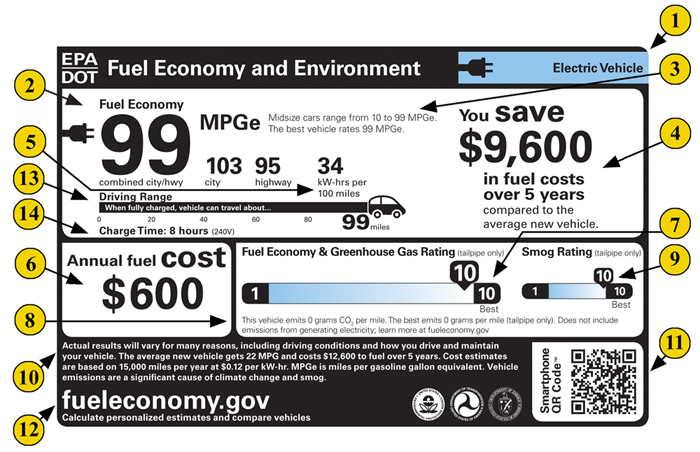# Calculator: MPGe to kWh

## MPGe to Energy Consumption and Fuel Equivalent Converter

The MPGe rating for an electric vehicle is often provided by the manufacturer or can be found on the vehicle's window sticker. You can also find this information in the U.S. Department of Energy's Fuel Economy website.

Enter MPGe (Miles Per Gallon equivalent) of your electric vehicle:

Use the ARBA Auto app to monitor the efficiency of your vehicle.

MPGe stands for "Miles Per Gallon Equivalent" (Wikipedia). This is a measure used in the United States to compare the energy consumption of alternative fuel vehicles, hybrid vehicles, and electric vehicles with the fuel economy of conventional internal combustion engine vehicles.

To understand MPGe, it's helpful to first understand MPG (miles per gallon), which is a common measure of how far a car can travel per gallon of gasoline. MPGe serves a similar purpose for electric vehicles, telling you how far the car can travel on the amount of energy that's equivalent to one gallon of gasoline.

To convert MPGe to kWh per 100 miles or kilometers, you can use the following steps:

1. Convert MPGe to energy consumption per mile by dividing 33.7 (the energy content in kWh of one gallon of gasoline) by the MPGe value. This gives you the energy consumption in kWh per mile.

2. Convert kWh per mile to kWh per 100 miles or kilometers. Multiply the kWh per mile value by 100 for miles, or divide it by 0.621371 for kilometers.

Remember, MPGe is a useful measure for comparing different vehicles, but it doesn't directly translate to the cost of driving an electric vehicle. This will depend on other factors, such as the cost of electricity in your area.